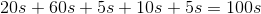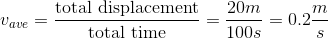# AP Physics C: Mechanics : Understanding Velocities

## Example Questions

### Example Question #1 : Understanding Velocities

The velocity (in meters per second) of a moving particle is given by the following function: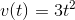If the particle's initial position is 0m, what is the position of the particle after two seconds?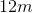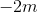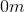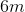Explanation:

To solve this problem you need to obtain a function of position with respect to time. For that, you need to understand velocity as the rate of change of displacement with respect to time. In other words, velocity is "how fast" (i.e. how much time it takes) an object changes position (remember displacement is the change in position). This means that velocity is the derivative of displacement with respect to time.

Therefore, to obtain a function of position with respect to time you need to take the antiderivative of the velocity function, so we integrate: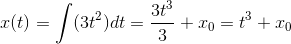Here,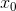is a constant that represents the initial position of the particle. We know that the initial position of the particle is 0m, so our function is: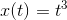Therefore, after two seconds have passed, we have t = 2s and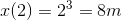Note: we know that position is given in meters since the question specified that velocity is measured in meters per second.

### Example Question #2 : Understanding Velocities

This morning you walked 20 meters to the right from your house to the bus stop, which took you 20 seconds. You waited at the bus stop for 1 minute before realizing you forgot your physics homework at home. You ran back to your house in 5 seconds. It took you 10 seconds to find your homework, and then you ran back to the bus stop in 5 seconds just in time to catch the bus. What was your average velocity for the entire period of motion?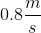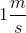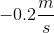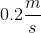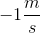Explanation:

This question tests your understanding of average velocity. You have different velocities throughout your motion: you changed direction and speed several times, including periods of no motion. However, average velocity cares only about your total displacement and how long it took you to get from your initial position to your final position.

Therefore, it is unnecessary to calculate your velocity at each time interval. You know your initial position is at your house and, after the entire motion, your final position is at the bus stop which is 20m to the right of your house. From this we get that the total displacement is 20m (note that it is positive because you moved to the right).

Since you moved back and forth and were motionless for some time intervals, it took you a total of 100s to finally get to the bus stop for good and catch the bus. You need to add all the times for each section of your motion; remember there are 60s in a minute and you need to be consistent with units.